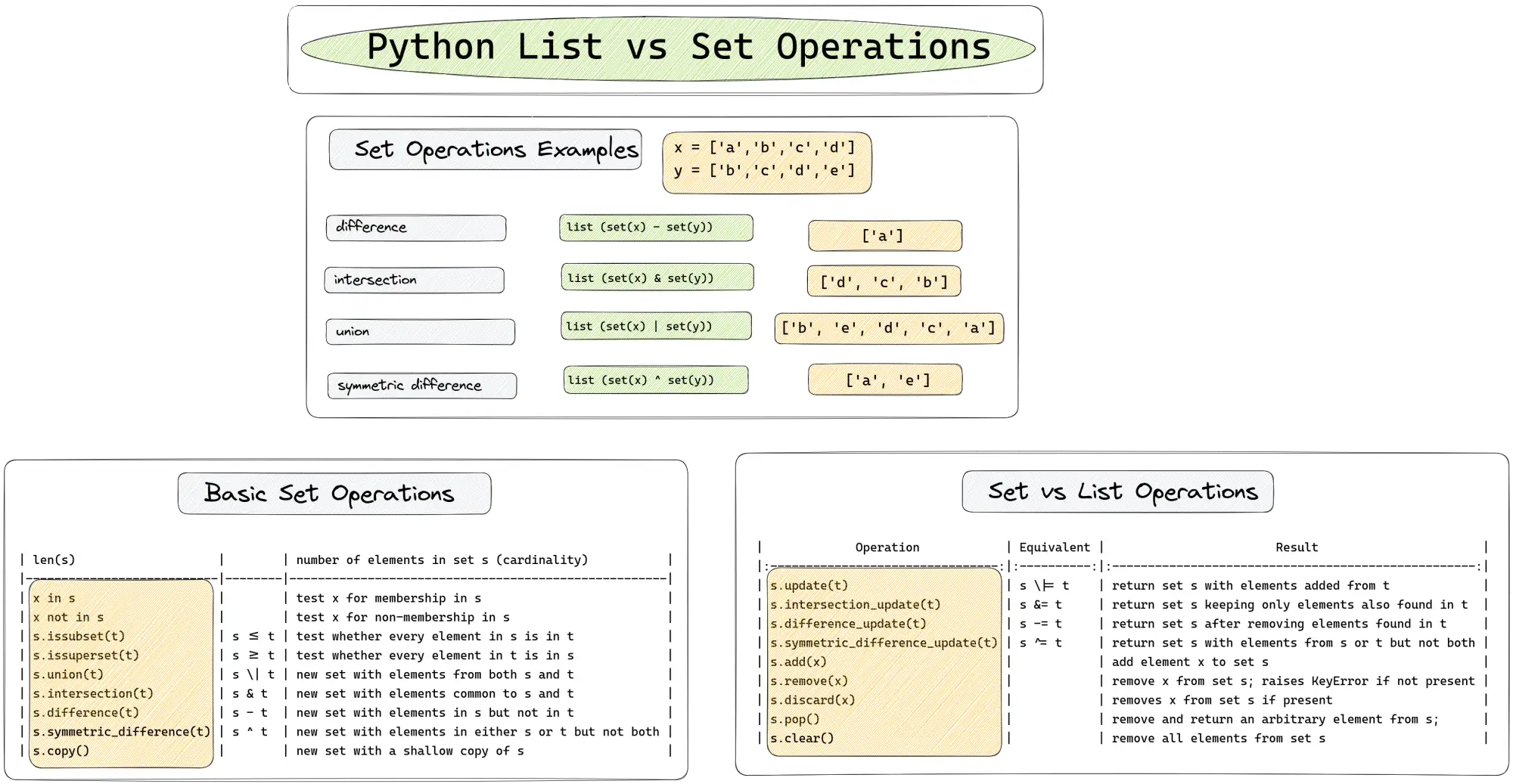In this article, we will compare and explain the most common list and set operation in Python. We will cover the advantages of using set and table with examples.## Why would you use a set?

Python offers set operations like:

• `intersection` - get elements from list1 and list2
• `union` - return all unique elements from both lists
• `union all` - all unique elements from both lists with repetition
• `subtraction/difference` - elements from list1 not in list2
• `symmetric_difference` - return a new set with elements in either the set or other but not both.

Sets also provide built-in methods for performing these operations. In some cases you may need to expand their behaviour.

## Set vs List in python

In Python

• `list` is an ordered collection of elements that can contain duplicates
• allow duplicates
• mutable
• `set` is an unordered collection of unique elements
• unique elements
• mutable
• better performance

Both data structures have their own advantages and use cases.

## Python list vs set performance

When it comes to performance, `set` have much better performance than lists. For certain operations like the `in` operator

• `set` - has O(1) average time complexity
• `list` - has O(n).

So checking if an element is present in a set is much faster than same check for a list.

• `union`
• `intersection`
• `difference`

also have better time complexity than their list counterparts.

Dealing with list with many elements then you need to use set to speed up your applications.

## Set operations Examples

`set` gives you an easy way to find the difference or the intersection of two lists.

In the next code examples we can see most common set operations:

``````x = ['a','b','c','d']
y = ['b','c','d','e']

print (list (set(x) - set(y))) # difference
print (list (set(y) - set(x)))
print (list (set(x) & set(y))) # intersection
print (list (set(x) | set(y))) # union
print (list (set(x) ^ set(y))) # symmetric_difference
``````

result:

``````x - y:   	['a']
y - x:   	['e']
x & y:   	['d', 'c', 'b']
x | y:   	['b', 'e', 'd', 'c', 'a']
x ^ y:   	['a', 'e']
``````

This example works with lists/sets from different sizes.

If you have interest take a look in this: Set Types — set, frozenset

## Python set operations

Below you can find table about most common set operations:

### Basic set operations

Operation Equivalent Result
len(s)   number of elements in set s (cardinality)
x in s   test x for membership in s
x not in s   test x for non-membership in s
s.issubset(t) s <= t test whether every element in s is in t
s.issuperset(t) s >= t test whether every element in t is in s
s.union(t) s | t new set with elements from both s and t
s.intersection(t) s & t new set with elements common to s and t
s.difference(t) s - t new set with elements in s but not in t
s.symmetric_difference(t) s ^ t new set with elements in either s or t but not both
s.copy()   new set with a shallow copy of s

## Python set operations on lists

Operation Equivalent Result
s.update(t) s |= t return set s with elements added from t
s.intersection_update(t) s &= t return set s keeping only elements also found in t
s.difference_update(t) s -= t return set s after removing elements found in t
s.symmetric_difference_update(t) s ^= t return set s with elements from s or t but not both
s.remove(x)   remove x from set s; raises `KeyError if not present`
s.pop()   remove and return an arbitrary element from s; raises `KeyError if empty`